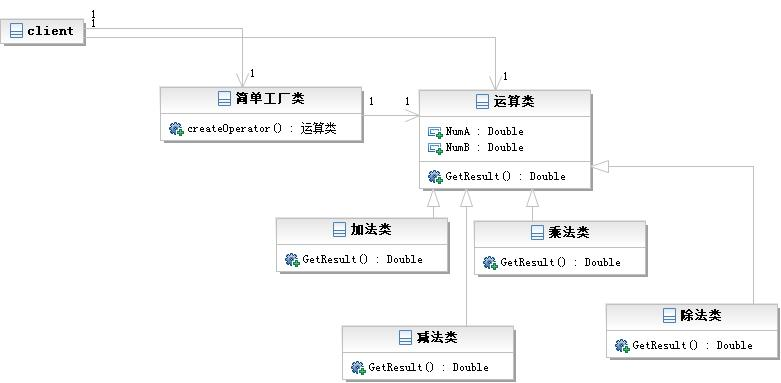# 使用简单工厂模式来进行Python的设计模式编程

piaodoo 编程教程 2020-02-02 12:34:40 188 0 python教程

```def create_animal(name):
if name == 'dog':
return Dog()
elif name == 'cat':
return Cat()

animal = create_animal('dog')
animal.eat('some food')

```

create_animal就是一个工厂，各种动物就是产品，该工厂根据name来决定产出什么动物产品。产品应该具有一个基本特性，同一个工厂出产的所有产品都是一个系列，都具有相同的功能，比如动物都吃食物。

```def create_operator(op):
if op == '+':
elif op == '-':
return SubOperation()
elif op == '*':
return MulOperation()
elif op == '/':
return DivOperation()

op = create_operator('+')
op.Calc(1, 2)

```

eg：采用简单工厂模式实现计算器

```#encoding=utf-8
#
#by panda
#简单工厂模式

class OperateBase():
result = 0;
def GetResult(self):
return self.result;

def __init__(self, NumA, NumB):
self.result = NumA + NumB;
def GetResult(self):
return self.result;

class OperationSub(OperateBase):
def __init__(self, NumA, NumB):
self.result = NumA - NumB;
def GetResult(self):
return self.result;

class OperationMult(OperateBase):
def __init__(self, NumA, NumB):
self.result = NumA * NumB;
def GetResult(self):
return self.result;

class OperationDiv(OperateBase):
def __init__(self, NumA, NumB):
self.result = NumA / NumB;
def GetResult(self):
return self.result;

class OperationFactory():
@staticmethod
def createOperate(operate, NumA, NumB):
optList = {
'-':OperationSub,
'*':OperationMult,
'/':OperationDiv,
}
oper = OperateBase()
if(optList.has_key(operate)):
oper = optList[operate](NumA, NumB);
return oper

def clientUI():
opt = raw_input("please input a operation(+-*/): ");
NumA = raw_input("please input the first number: ");
NumB = raw_input("please input the second number: ");
oper = OperationFactory.createOperate(opt, float(NumA), float(NumB))
print "Restlt: ", oper.GetResult()
return

if __name__ == '__main__':
clientUI();
```

UML类图如下：